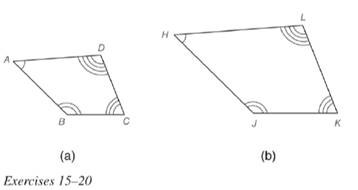Chapter 5.2, Problem 15EElementary Geometry For College St...

7th Edition
Alexander + 2 others
ISBN: 9781337614085

Solutions

Chapter
SectionElementary Geometry For College St...

7th Edition
Alexander + 2 others
ISBN: 9781337614085
Textbook Problem

Quadrilateral A B C D ∼ quadrilateral H J K L . If m ∠ A = 55 ∘ , m ∠ J = 128 ∘ and m ∠ D = 98 ∘ , find m ∠ K .To determine

To calculate:

The value of mK.

Explanation

Given:

mA=55,mJ=128andmD=98.

Definition Used:

The sum of measure of angle in a quadrilateral is 360.

Similarity between Two polygons:

Two polygons are similar if and only if when all pairs of corresponding angles are congruent and all pairs of corresponding sides are proportional.

Calculation:

According to the definition of similar polygon, AH,BJ,DL,andCK

Here, congruent angles are of same measure.

Still sussing out bartleby?

Check out a sample textbook solution.

See a sample solution

The Solution to Your Study Problems

Bartleby provides explanations to thousands of textbook problems written by our experts, many with advanced degrees!

Get Started

let f(x) = x3 + 5, g(x) = x2 2, and h(x)= 2x + 4. Find the rule for each function. 5. fg

Applied Calculus for the Managerial, Life, and Social Sciences: A Brief Approach

Evaluate the integral. 43. x1+x3dx

Single Variable Calculus: Early Transcendentals

Study Guide for Stewart's Multivariable Calculus, 8th

f(x) = 12x x3 has a local maximum at: a) x = 0 b) x = 2 c) x = 2 d) f does not have a local maximum

Study Guide for Stewart's Single Variable Calculus: Early Transcendentals, 8th